Multi-Dimensional Packet Classiﬁcation:Classiﬁcation Algorithms

Classiﬁcation Algorithms

Background

For the next few sections, we will use the example classiﬁer in Table 49.5 repeatedly.

The classiﬁer has six rules in two ﬁelds labeled F 1 and F 2; each speciﬁcation is a preﬁx of maximum length 3 bits. We will refer to the classiﬁer as C = {Rj } and each rule Rj as a 2-tuple: < Rj1, Rj2 >.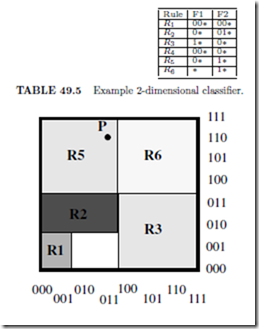FIGURE 49.3: Geometric representation of the classiﬁer in Table 49.5. A packet represents a point, for instance P(011,110) in two-dimensional space. Note that R4 is hidden by R1 and R2.

Bounds from Computational Geometry

There is a simple geometric interpretation of packet classiﬁcation. While a preﬁx represents a contiguous interval on the number line, a two-dimensional rule represents a rectangle in two-dimensional euclidean space, and a rule in d dimensions represents a d-dimensional hyper-rectangle. A classiﬁer is therefore a collection of prioritized hyper-rectangles, and a packet header represents a point in d dimensions. For example, Figure 49.3 shows the classiﬁer in Table 49.5 geometrically in which high priority rules overlay lower priority rules. Classifying a packet is equivalent to ﬁnding the highest priority rectangle that contains the point representing the packet. For example, point P(011,110) in Figure 3 would be classiﬁed by rule R5.

There are several standard geometry problems such as ray shooting, point location and rectangle enclosure that resemble packet classiﬁcation. Point location involves ﬁnding the enclosing region of a point, given a set of non-overlapping regions. The best bounds for point location in N rectangular regions and d > 3 dimensions are O(log N ) time with O(N d) space;or O((log N )d1) time with O(N ) space [8, 9]. In packet classiﬁcation, hyper- rectangles can overlap, making classiﬁcation at least as hard as point location. Hence, a solution is either impracticably large (with 100 rules and 4 ﬁelds, N d space is about 100MBytes) or too slow ((log N )d1 is about 350 memory accesses).

We can conclude that: (1) Multi-ﬁeld classiﬁcation is considerably more complex than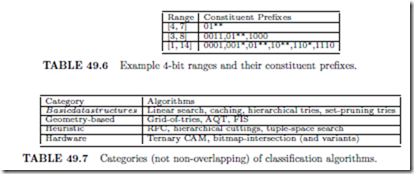one-dimensional longest preﬁx matching, and (2) Complexity may require that practical solutions use heuristics.

Range lookups

Packet classiﬁcation is made yet more complex by the need to match on ranges as well as preﬁxes. The cases of looking up static arbitrary ranges, and dynamic conﬂict-free ranges in one dimension have been discussed in Chapter 48.

One simple way to handle dynamic arbitrary (overlapping) ranges is to convert each W -bit range to a set of 2W 2 preﬁxes (see Table 49.6) and then use any of the longest preﬁx matching algorithms detailed in Chapter 48, thus resulting in O(NW ) preﬁxes for a set consisting of N ranges.

Taxonomy of Classiﬁcation Algorithms

The classiﬁcation algorithms we will describe here can be categorized into the four classes shown in Table 49.7.

We now proceed to describe representative algorithms from each class.

Basic Data Structures

Linear search

The simplest data structure is a linked-list of rules stored in order of decreasing priority. A packet is compared with each rule sequentially until a rule is found that matches all relevant ﬁelds. While simple and storage-eﬃcient, this algorithm clearly has poor scaling properties; the time to classify a packet grows linearly with the number of rules.

Hierarchical tries

A d-dimensional hierarchical radix trie is a simple extension of the one dimensional radix trie data structure, and is constructed recursively as follows. If d is greater than 1, we ﬁrst construct a 1-dimensional trie, called the F 1-trie, on the set of preﬁxes {Rj1}, belonging to dimension F 1 of all rules in the classiﬁer, C = {Rj } = {< Rj1 , Rj2,... , Rjd >}. For each preﬁx, p, in the F 1-trie, we recursively construct a (d 1)-dimensional hierarchical trie, Tp, on those rules which specify exactly p in dimension F 1, i.e., on the set of rules {Rj : Rj1 = p}. Preﬁx p is linked to the trie Tp using a next-trie pointer. The storage complexity of the data structure for an N -rule classiﬁer is O(N dW ). The data structure for the classiﬁer in Table 49.5 is shown in Figure 49.4. Hierarchical tries are sometimes called “multi-level tries,” “backtracking-search tries,” or “trie-of-tries”.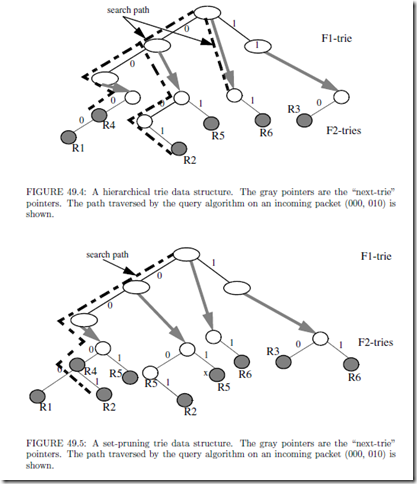Classiﬁcation of an incoming packet (v1, v2,…, vd) proceeds as follows. The query algo- rithm ﬁrst traverses the F 1-trie based on the bits in v1. At each F 1-trie node encountered, the algorithm follows the next-trie pointer (if present) and traverses the (d 1)-dimensional trie. The query time complexity for d-dimensions is therefore O(W d ). Incremental updates can be carried out similarly in O(d2 W ) time since each component of the updated rule is stored in exactly one location at maximum depth O(dW ).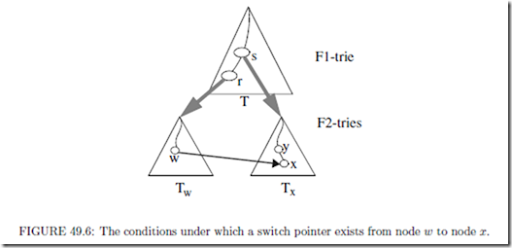Set-pruning tries

A set-pruning trie data structure  is similar, but with reduced query time obtained by replicating rules to eliminate recursive traversals. The data structure for the classiﬁer in Ta- ble 49.5 is shown in Figure 49.5. The query algorithm for an incoming packet (v1, v2,... , vd) need only traverse the F 1-trie to ﬁnd the longest matching preﬁx of v1, follow its next-trie pointer (if present), traverse the F 2-trie to ﬁnd the longest matching preﬁx of v1, and so on for all dimensions. The rules are replicated to ensure that every matching rule will be encountered in the path. The query time is reduced to O(dW ) at the expense of increased storage of O(N ddW ) since a rule may need to be replicated O(N d ) times. Update com- plexity is O(N d), and hence, this data structure works only for relatively static classiﬁers.

Geometric Algorithms

Grid-of-tries

The grid-of-tries data structure, proposed by Srinivasan et al  for 2-dimensional classiﬁcation, reduces storage space by allocating a rule to only one trie node as in a hierarchical trie, and yet achieves O(W ) query time by pre-computing and storing a switch pointer in some trie nodes. A switch pointer is labeled with ‘0’ or ‘1’ and guides the search process. The conditions which must simultaneously be satisﬁed for a switch pointer labeled b (b = ’0’ or ‘1’) to exist from a node w in the trie Tw to a node x of another trie Tx are (see Figure 49.6):

1. Tx and Tw are distinct tries built on the preﬁx components of dimension F 2. Tx and Tw are pointed to by two distinct nodes, say r and s respectively of the same trie, T , built on preﬁx components of dimension F 1.

2. The bit-string that denotes the path from the root node to node w in trie Tw concatenated with the bit b is identical to the bit-string that denotes the path from the root node to node x in the trie Tx.

3. Node w does not have a child pointer labeled b, and

4. Node s in trie T is the closest ancestor of node r that satisﬁes the above conditions.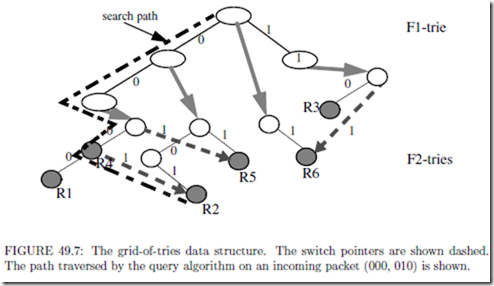If the query algorithm traverses paths U 1(s, root(Tx), y, x) and U 2(r, root(Tw ), w) in a hierarchical trie, it need only traverse the path (s, r, root(Tw ), w, x) on a grid-of-tries. This is because paths U 1 and U 2 are identical (by condition 2 above) till U 1 terminates at node w because it has no child branch (by condition 3 above). The switch pointer eliminates the need for backtracking in a hierarchical trie without the storage overhead of a set-pruning trie. Each bit of the packet header is examined at most once, so the time complexity reduces to O(W ), while storage complexity O(NW ) is the same as a 2-dimensional hierarchical trie. However, the presence of switch pointers makes incremental updates diﬃcult, so the authors recommend rebuilding the data structure (in time O(NW )) for each update. An example of the grid-of-tries data structure is shown in Figure 49.7.

Reference  reports 2MBytes of storage for a 20,000 two-dimensional classiﬁer with destination and source IP preﬁxes in a maximum of 9 memory accesses.

Grid-of-tries works well for two dimensional classiﬁcation, and can be used for the last two dimensions of a multi-dimensional hierarchical trie, decreasing the classiﬁcation time complexity by a factor of W to O(NW d1). As with hierarchical and set-pruning tries, rid-of-tries handles range speciﬁcations by splitting into preﬁxes.

Cross-producting

Cross-producting  is suitable for an arbitrary number of dimensions. Packets are classiﬁed by composing the results of separate 1-dimensional range lookups for each dimension as explained below.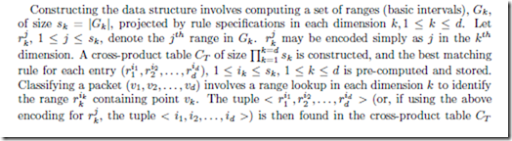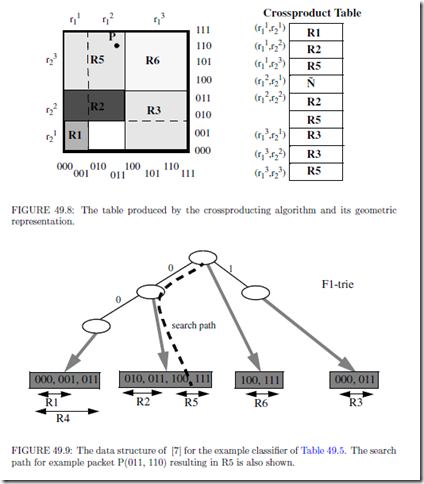which contains the pre-computed best matching rule. Figure 49.8 shows an example. Given that N preﬁxes lead to at most 2N 2 ranges, sk 2N and CT is of size O(N d).

The lookup time is O(dtRL ) where tRL is the time complexity of ﬁnding a range in one dimension. Because of its high worst case storage complexity, cross-producting is suitable only for very small classiﬁers. Reference  proposes using an on-demand cross-producting scheme together with caching for classiﬁers bigger than 50 rules in ﬁve dimensions. Updates require reconstruction of the cross-product table, and so cross-producting is suitable for relatively static classiﬁers.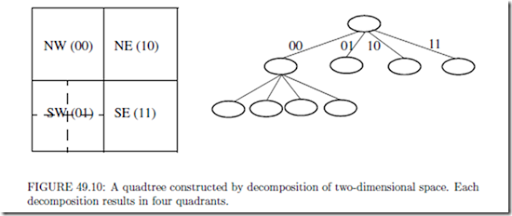A 2-dimensional classiﬁcation scheme 

Lakshman and Stiliadis  propose a 2-dimensional classiﬁcation algorithm where one dimension, say F 1, is restricted to have preﬁx speciﬁcations while the second dimension, F 2, is allowed to have arbitrary range speciﬁcations. The data structure ﬁrst builds a trie on the preﬁxes of dimension F 1, and then associates a set Gw of non-overlapping ranges to each trie node, w, that represents preﬁx p. These ranges are created by (possibly overlapping) projections on dimension F 2 of those rules, Sw , that specify exactly p in dimension F 1. A range lookup data structure (e.g., an array or a binary search tree) is then constructed on Gw and associated with trie node w. An example is shown in Figure 49.9.

Searching for point P (v1, v2) involves a range lookup in data structure Gw for each trie node, w, encountered. The search in Gw returns the range containing v2, and hence the best matching rule. The highest priority rule is selected from the rules {Rw } for all trie nodes encountered during the traversal.

The storage complexity is O(NW ) because each rule is stored only once in the data structure. Queries take O(W log N ) time because an O(log N ) range lookup is performed for every node encountered in the F 1-trie. This can be reduced to O(W + log N ) using fractional cascading , but that makes incremental updates impractical.

Two instances of the same data structure are associated with each quadtree node —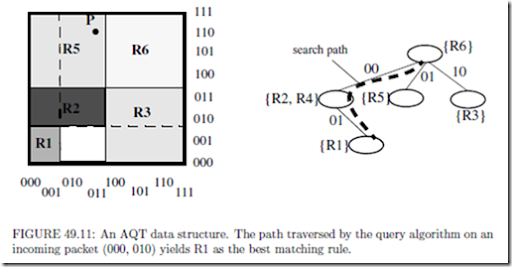each stores the rules in k-CFS (k=1,2). Since rules in crossing ﬁlter sets span at least one dimension, only the range speciﬁed in the other dimension need be stored. Queries proceed two bits at a time by transposing one bit from each dimension, with two 1-dimensional lookups being performed (one for each dimension on k-CFS) at each node.

shows an example.

Figure 49.11

Reference  proposes an eﬃcient update algorithm that, for N two-dimensional rules, has O(NW ) space complexity, O(αW ) search time and O(α α N ) update time, where α is a tunable integer parameter.

Fat Inverted Segment tree (FIS-tree)

Feldman and Muthukrishnan  propose the Fat Inverted Segment tree (FIS-tree) for two dimensional classiﬁcation as a modiﬁcation of a segment tree. A segment tree  stores a set S of possibly overlapping line segments to answer queries such as ﬁnding the highest priority line segment containing a given point. A segment tree is a balanced binary search tree containing the end points of the line segments in S. Each node, w, represents a range Gw , the leaves represent the original line segments in S, and parent nodes represent the union of the ranges represented by their children. A line segment is allocated to a node w if it contains Gw but not Gparent(w) . The highest priority line segment allocated to a node is pre-computed and stored at the node. A query traverses the segment tree from the root, calculating the highest priority of all the pre-computed segments encountered. Figure 49.12 shows an example segment tree.

An FIS-tree is a segment tree with two modiﬁcations: (1) The segment tree is compressed (made “fat” by increasing the degree to more than two) in order to decrease its depth and occupies a given number of levels l, and (2) Up-pointers from child to parent nodes are used. The data structure for 2-dimensions consists of an FIS-tree on dimension F 1 and a range lookup data associated with each node. An instance of the range lookup data structure associated with node w of the FIS-tree stores the ranges formed by the F 2-projections of those classiﬁer rules whose F 1-projections were allocated to w.

A query for point P (v1, v2) ﬁrst solves the range lookup problem on dimension F 1. This returns a leaf node of the FIS-tree representing the range containing the point v1. The query algorithm then follows the up-pointers from this leaf node towards the root node, carrying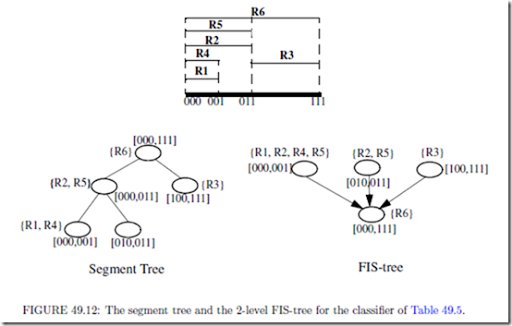out 1-dimensional range lookups at each node. The highest priority rule containing the given point is calculated at the end of the traversal.

Queries on an l-level FIS-tree have complexity O((l + 1)tRL) with storage complexity O(ln1+1/l ), where tRL is the time for a 1-dimensional range lookup. Storage space can be traded oﬀ with search time by varying l. Modiﬁcations to the FIS-tree are necessary to support incremental updates — even then, it is easier to support inserts than deletes . The static FIS-tree can be extended to multiple dimensions by building hierarchical FIS- trees, but the bounds are similar to other methods studied earlier .

Measurements on real-life 2-dimensional classiﬁers are reported in  using the static FIS-tree data structure. Queries took 15 or fewer memory operations with a two level tree, 4-60K rules and 5MBytes of storage. Large classiﬁers with one million 2-dimensional rules required 3 levels, 18 memory accesses per query and 100MBytes of storage.

Dynamic multi-level tree algorithms

Two algorithms, called Heap-on-Trie (HoT) and Binarysearchtree-on-Trie (BoT) are introduced in  that build a heap and binary search tree respectively on the last dimension, and multi-level tries on all the remaining d 1 dimensions. If W = O(log N ): HoT has query complexity O(logd N ), storage complexity O(N logd N ), and update complexity O(logd+1 N ); and BoT has query complexity O(logd+1 N ), storage complexity O(N logd N ), and update complexity O(logd N ). If W j= O(log N ), each of the above complexity formulae need to be modiﬁed to replace a factor O(logd1 N ) with O(W d1).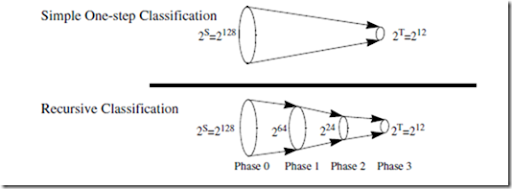FIGURE 49.13: Showing the basic idea of Recursive Flow Classiﬁcation. The reduction is carried out in multiple phases, with a reduction in phase I being carried out recursively on the image of the phase I 1. The example shows the mapping of 2S bits to 2T bits in three phases.

Heuristics

As we saw in Section 49.3.1, the packet classiﬁcation problem is expensive to solve in the worst-case — theoretical bounds state that solutions to multi-ﬁeld classiﬁcation either require storage that is geometric, or a number of memory accesses that is polylogarithmic, in the number of classiﬁcation rules. We can expect that classiﬁers in real networks have considerable structure and redundancy that might be exploited by a heuristic. That is the motivation behind the algorithms described in this section.

Recursive Flow Classiﬁcation (RFC)

RFC  is a heuristic for packet classiﬁcation on multiple ﬁelds. Classifying a packet involves mapping S bits in the packet header to a T bit action identiﬁer, where T = log N, T « S.

A simple, but impractical method could pre-compute the action for each of the 2S diﬀerent packet headers, yielding the action in one step. RFC attempts to perform the same mapping over several phases, as shown in Figure 49.13; at each stage the algorithm maps one set of values to a smaller set. In each phase a set of memories return a value shorter (i.e., expressed in fewer bits) than the index of the memory access. The algorithm, illustrated in Figure 49.14, operates as follows:

1. In the ﬁrst phase, d ﬁelds of the packet header are split up into multiple chunks that are used to index into multiple memories in parallel. The contents of each memory are chosen so that the result of the lookup is narrower than the index.

2. In subsequent phases, memories are indexed using the results from earlier phases.

3. In the ﬁnal phase, the memory yields the action.

The algorithm requires construction of the contents of each memory detailed in . This paper reports that with real-life four-dimensional classiﬁers of up to 1,700 rules, RFC ap- pears practical for 10Gbps line rates in hardware and 2.5Gbps rates in software. However, the storage space and pre-processing time grow rapidly for classiﬁers larger than 6,000 rules. An optimization described in  reduces the storage requirement of a 15,000 four-ﬁeld classiﬁer to below 4MBytes.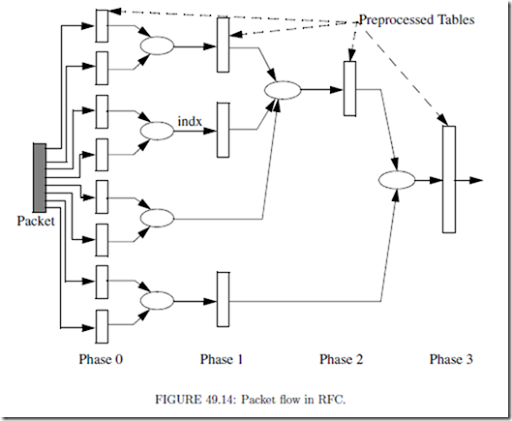Hierarchical Intelligent Cuttings (HiCuts)

HiCuts  partitions the multi-dimensional search space guided by heuristics that exploit the structure of the classiﬁer. Each query leads to a leaf node in the HiCuts tree, which stores a small number of rules that can be searched sequentially to ﬁnd the best match. The characteristics of the decision tree (its depth, degree of each node, and the local search decision to be made at each node) are chosen while pre-processing the classiﬁer based on its characteristics (see  for the heuristics used).

Each node, v, of the tree represents a portion of the geometric search space. The root node represents the complete d-dimensional space, which is partitioned into smaller geometric sub-spaces, represented by its child nodes, by cutting across one of the d dimensions. Each sub-space is recursively partitioned until no sub-space has more than B rules, where B is a tunable parameter of the pre-processing algorithm. An example is shown in Figure 49.15 for two dimensions with B = 2.

Parameters of the HiCuts algorithm can be tuned to trade- oﬀ query time against storage requirements. On 40 real-life four-dimensional classiﬁers with up to 1,700 rules, HiCuts requires less than 1 MByte of storage with a worst case query time of 20 memory accesses, and supports fast updates.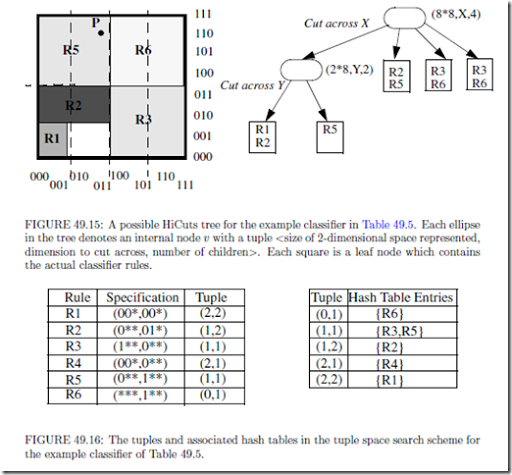Tuple Space Search

The basic tuple space search algorithm  decomposes a classiﬁcation query into a number of exact match queries. The algorithm ﬁrst maps each d-dimensional rule into a d-tuple whose ith component stores the length of the preﬁx speciﬁed in the ith dimension of the rule (the scheme supports only preﬁx speciﬁcations). Hence, the set of rules mapped to the same tuple are of a ﬁxed and known length, and can be stored in a hash table. Queries perform exact match operations on each of the hash tables corresponding to all possible tuples in the classiﬁer. An example is shown in Figure 49.16.

Query time is M hashed memory accesses, where M is the number of tuples in the classiﬁer. Storage complexity is O(N ) since each rule is stored in exactly one hash table. Incremental updates are supported and require just one hashed memory access to the hashed table associated with the tuple of the modiﬁed rule. In summary, the tuple space search algorithm performs well for multiple dimensions in the average case if the number of tuples is small. However, the use of hashing makes the time complexity of searches and updates non-deterministic. The number of tuples could be very large, up to O(W d ), in the worst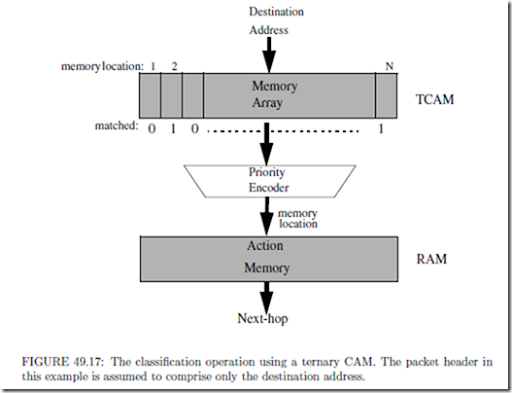case. Furthermore, since the scheme supports only preﬁxes, the storage complexity increases by a factor of O(W d) for generic rules as each range could be split into W preﬁxes in the manner explained in Section 49.3.1. Of course, the algorithm becomes attractive for real-life classiﬁers that have a small number of tuples.

Hardware-Based Algorithms

Ternary CAMs

A TCAM stores each W -bit ﬁeld as a (val, mask) pair; where val and mask are each W -bit numbers. A mask of ‘0’ wildcards the corresponding bit position. For example, if W = 5, a preﬁx 10* will be stored as the pair (10000, 11000). An element matches a given input key by checking if those bits of val for which the mask bit is ‘1’, match those in the key.

A TCAM is used as shown in Figure 49.17. The TCAM memory array stores rules in decreasing order of priorities, and compares an input key against every element in the array in parallel. The N -bit bit-vector, matched, indicates which rules match and so the N -bit priority encoder indicates the address of the highest priority match. The address is used to index into a RAM to ﬁnd the action associated with this preﬁx. TCAMs are being increasingly deployed because of their simplicity of use and speed (as they are able to do classiﬁcation in hardware at the rate of the hardware clock).

Several companies today ship 9Mb TCAMs capable of single and multi-ﬁeld classiﬁcation in as little as 10ns. Both faster and denser TCAMs can be expected in the near future. There are, however, some disadvantages to TCAMs:

Handbook of Data Structures and Applications

1. A TCAM is less dense than a RAM, storing fewer bits in the same chip area.

One bit in an SRAM typically requires 4-6 transistors, while one bit in a TCAM requires 11-15 transistors . A 9Mb TCAM running at 100 MHz costs about \$200 today, while the same amount of SRAM costs less than \$10. Furthermore, range speciﬁcations need to be split into multiple masks, reducing the number of entries by up to (2W 2)d in the worst case. If only two 16-bit dimensions specify ranges, this is a multiplicative factor of 900. Newer TCAMs, based on DRAM technology, have been proposed and promise higher densities.

2. TCAMs dissipate more power than RAM solutions because an address is com- pared against every TCAM element in parallel. At the time of writing, a 9Mb TCAM chip running at 100 MHz dissipates about 10-15 watts (the exact number varies with manufacturer). In contrast, a similar amount of SRAM running at the same speed dissipates 1W.

3. A TCAM is more unreliable while being in operational use in a router in the ﬁeld than a RAM, because a soft-error (error caused by alpha particles and package impurities that can ﬂip a bit of memory from 0 to 1, or vice-versa) could go undetected for a long amount of time. In a SRAM, only one location is accessed at any time, thus enabling easy on-the-ﬂy error detection and correction. In a TCAM, wrong results could be given out during the time that the error is undetected – which is particularly problematic in such applications as ﬁltering or security.

However, the above disadvantages of a TCAM need to be weighed against its enormous simplicity of use in a hardware platform. Besides, it is the only known “algorithm” that is capable of doing classiﬁcation at high speeds without explosion in either storage space or time.

Due to their high cost and power dissipation, TCAMs will probably remain unsuitable in the near future for (1) Large classiﬁers (512K-1M rules) used for microﬂow recognition at the edge of the network, (2) Large classiﬁers (256-512K rules) used at edge routers that manage thousands of subscribers (with a few rules per subscriber). Of course, software- based routers need to look somewhere else.

Bitmap-intersection

The bitmap-intersection classiﬁcation scheme, proposed in , is based on the observation that the set of rules, S, that match a packet is the intersection of d sets, Si, where Si is the set of rules that match the packet in the ith dimension alone. While cross-producting pre-computes S and stores the best matching rule in S, this scheme computes S and the best matching rule during each classiﬁcation operation.

In order to compute intersection of sets in hardware, each set is encoded as an N -bit bitmap where each bit corresponds to a rule. The set of matching rules is the set of rules whose corresponding bits are ‘1’ in the bitmap. A query is similar to cross-producting: First, a range lookup is performed in each of the d dimensions. Each lookup returns a bitmap representing the matching rules (pre-computed for each range) in that dimension. The d sets are intersected (a simple bit-wise AND operation) to give the set of matching rules, from which the best matching rule is found. See Figure 49.18 for an example.

Since each bitmap is N bits wide, and there are O(N ) ranges in each of d dimensions, the storage space consumed is O(dN 2). Query time is O(dtRL + dN/w) where tRL is the time to do one range lookup and w is the memory width. Time complexity can be reduced by a factor of d by looking up each dimension independently in parallel. Incremental updates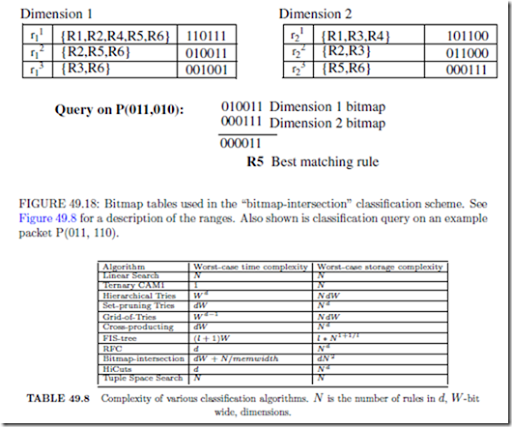are not supported.

Reference  reports that the scheme can support up to 512 rules with a 33 MHz ﬁeld- programmable gate array and ﬁve 1Mbit SRAMs, classifying 1Mpps. The scheme works well for a small number of rules in multiple dimensions, but suﬀers from a quadratic increase in storage space and linear increase in classiﬁcation time with the size of the classiﬁer. A variation is described in  that decreases storage at the expense of increased query time. This work has been extended signiﬁcantly by  by aggregating bitmaps wherever possible and thus decreasing the time spent in reading the bitmaps. Though the bitmap-intersection scheme is primarily meant for hardware, it is easy to see how it can be used in software, where the aggregated bitmap technique of  could be especially useful.